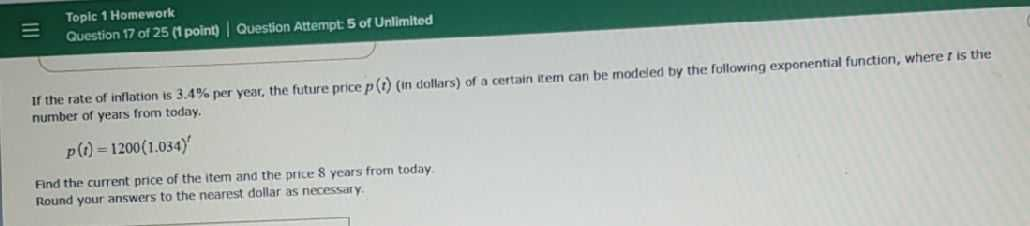### ¿Todavía tienes preguntas de matemáticas?

Pregunte a nuestros tutores expertos
Algebra
Pregunta$$=$$ Topic $$1$$ Homework If the rate of inflation is $$3.4 \%$$ per year, the future price $$p ( t )$$ (in dollars) of a certain item can be modeled by the following exponential function, where $$t$$ is the number of years from today. $$p ( t ) = 1200 ( 1.034 ) ^ { t }$$ .

Fnd the current price of the item and the price $$8$$ years from today. Round your answers to the nearest dollar as necessiry.